# COUNTA Function

Calculates the number of non-blank cells within a given set of values

## What is COUNTA (Excel Countif Not Blank)?

The COUNTA Function is categorized under Excel Statistical functions. It will calculate the number of cells that are not blank within a given set of values.  The COUNTA function is also commonly referred to as the Excel COUNTIF Not Blank formula.

As a financial analyst, the COUNTA function is useful if we wish to keep a count of cells in a given range. Apart from crunching numbers, we often need to count cells with values. In such a scenario, the function can be useful.

### Excel Countif Not Blank Formula

=COUNTA(value1, [value2], …)

The Excel countif not blank formula uses the following arguments:

1. Value1 (required argument) – This is the value at which we evaluate the function.
2. Value2 (optional argument) – Additional arguments that represent the values that we wish to count.

#### A few notes about the arguments

1. We can enter up to 255 value arguments if we are using MS Excel 2007 or later. Earlier versions can handle 30 arguments only.
2. Value arguments can be values, an array of values, or references to cell ranges.

### How to use the COUNTA Function in Excel?

To understand the uses of the COUNTA function, let’s consider a few examples:

#### Example 1 – Excel Countif not blank

Suppose we are given the data below: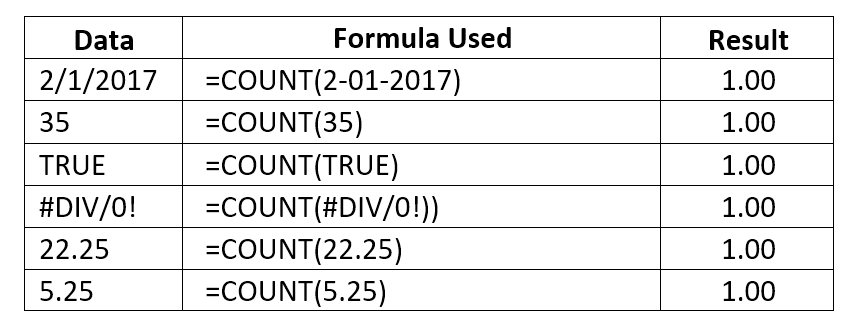As seen above, the COUNTA function will count text or formula errors. So unlike the COUNT function, which considers only numbers, COUNTA considers numbers, dates, text values, logical values, and errors.

We get the results below: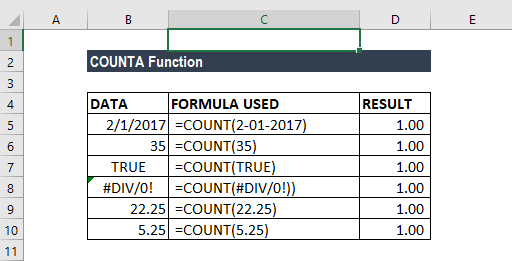The COUNTA function can be used for an array. If we enter the formula =COUNTA(B5:B10), we will get the result 6, as shown below:

#### Example 2 – Excel Countif not blank

Suppose we wish to count the number of cells that contain data in a given set, as shown below: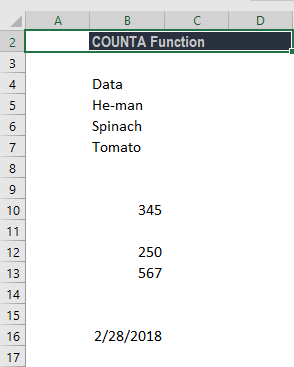To count the cells with data, we will use the formula =COUNTA(B4:B16).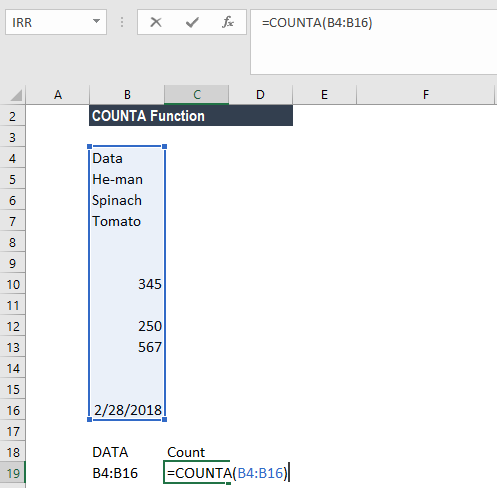We get 8 as the result, as the COUNTA function will not count cells that are absolutely empty cells.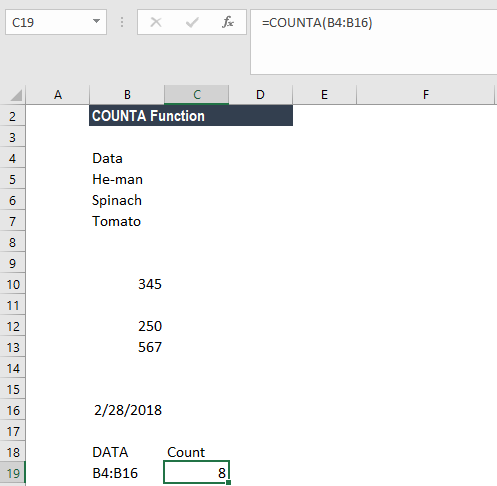#### Example 3 – Excel Countif function

Suppose we wish to count cells that are not equal to a range of certain things. We can use a combination of the COUNTA, COUNTIF, and SUMPRODUCT functions to get the desired results.

Suppose we are given the data below: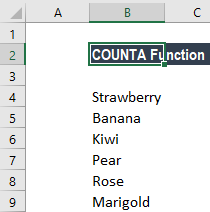To count cells not equal to Rose and Marigold, we used the following formula: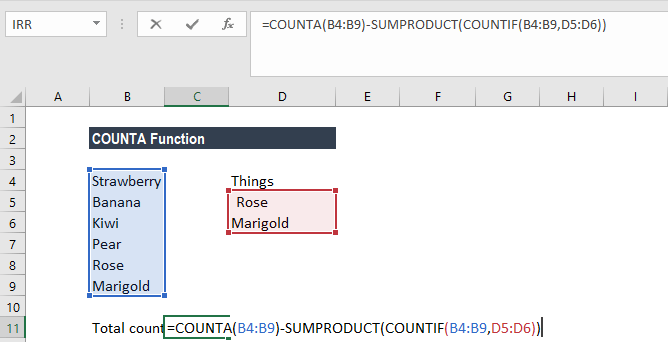We can list down the things we wish to exclude from counting. One other way to arrive at the same result is to use the formula =COUNTIFS(B4:B9,”<>Rose”B4:B9,”<>Marigold”).

However, the above formula would be tedious to use if the list contains many items. We need to add an additional range/criteria pair for each item that we don’t wish to count.

Hence, we can use a reference list and use the =COUNTA(B4:B9)-SUMPRODUCT(COUNTIF(B4:B9,D5:D6)) formula.

The formula uses the range D5:D6 to hold values that we don’t want to count. The formula starts by counting all values in the range being counted with COUNTA. Next, it generates a count of all things we don’t want to count with COUNTIF. Lastly, SUMPRODUCT will sum up all items in the array, which returns 2.  The number is then subtracted from the original total to yield the final result.

We get the result below: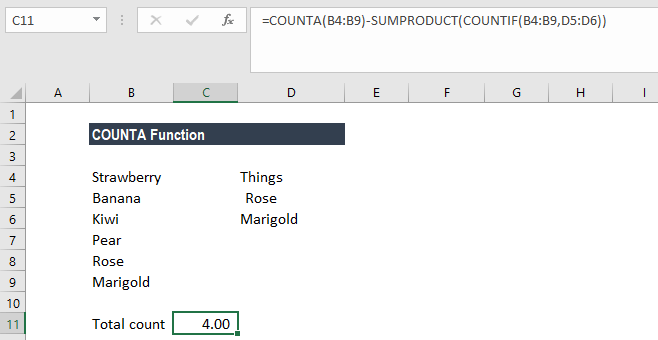### Things to remember about the COUNTA Function

1. If we wish to count logical values, we should use the COUNTA function, but if we want to count only cells that contain a number, we should use COUNT function.
2. The function belongs to the COUNT function family. There are five variants of COUNT functions: COUNT, COUNTA, COUNTBLANK, COUNTIF, and COUNTIFS.
3. We need to use the COUNTIF function or COUNTIFS function if we want to count only numbers that meet specific criteria.
4. If we wish to count based on certain criteria, we should use COUNTIF.

Thanks for reading CFI’s guide to important Excel functions! By taking the time to learn and master these functions, you’ll significantly speed up your financial analysis. To learn more, check out these additional CFI resources:

• What is Financial Modeling?
• Types of Financial Models
• Types of Graphs
• Financial Analyst Designation

### Free Excel Tutorial

To master the art of Excel, check out CFI’s FREE Excel Crash Course, which teaches you how to become an Excel power user.  Learn the most important formulas, functions, and shortcuts to become confident in your financial analysis.

Launch CFI’s Free Excel Course now to take your career to the next level and move up the ladder!